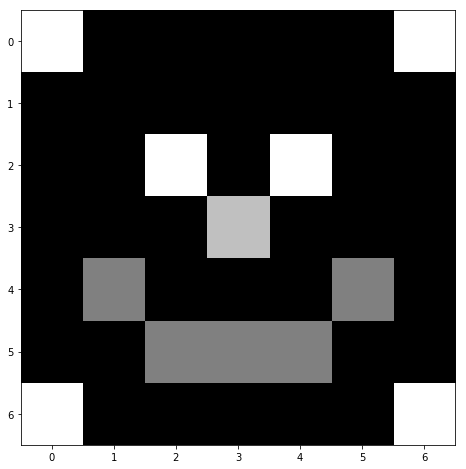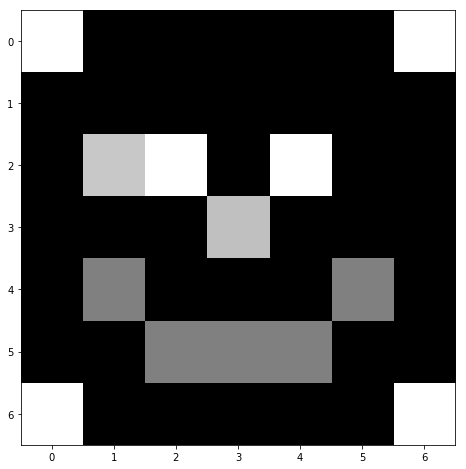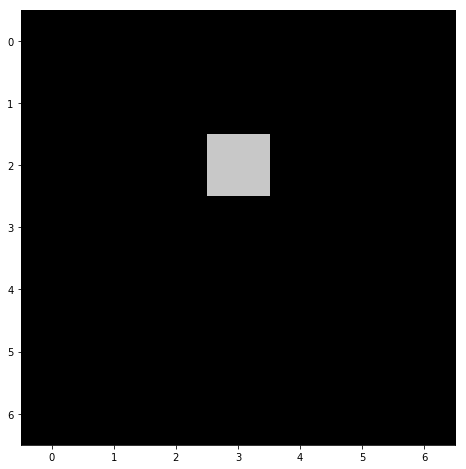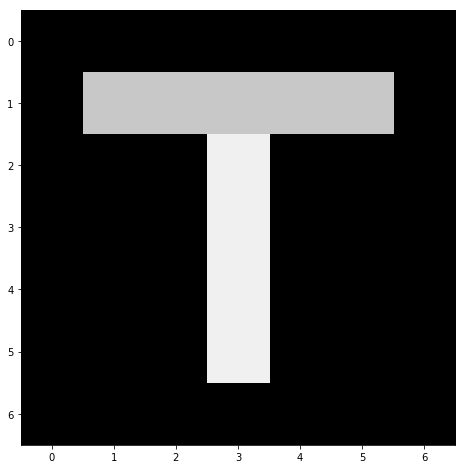# Part 1: Working with images¶

Welcome to the first workshop session of the AI-Radiology masterclass.

Today, we will review the basics of image processing for computational anatomy as we learn:

This web-based Jupyter notebook is meant to be interactive and user-friendly. Feel free to type your own code in the cells, and to run it using "Ctrl+Enter" or the toolbar's "Run" button.

N.B.: The Python syntax used here should be simple enough for you to follow this workshop session without hassle. Comments, written at the end of each line after the # symbol, describe in simple words the intention behind the code. If you don't understand something, "google is your friend"... And I'm here to answer your questions!

### First steps with Python¶

Out-of-the-box, the Python language is only equipped with text processing routines. Before going any further, we thus need to import high-level tools using the following syntax:

In :
%matplotlib inline
import center_images             # Center our images
import matplotlib.pyplot as plt  # Graphical display
import numpy as np               # Numerical computations


Thanks to the Python keyword import, we can now use :

• plt = plot curves and images,
• np = numeric computations with python,
• imread = image reader,

as new keywords in the cells below. For instance, the proper syntax to read images from the current folder reads:

In :
I = imread("data/smiley.png", as_gray=True)  # Import a png image as a grayscale array


As far as Python is concerned, I now denotes a variable that can be displayed, modified and saved. For instance, the print(...) function allows us to display variables as text:

In :
print(I)  # Print the variable 'I' in the space below:

[[255.   0.   0.   0.   0.   0. 255.]
[  0.   0.   0.   0.   0.   0.   0.]
[  0.   0. 255.   0. 255.   0.   0.]
[  0.   0.   0. 192.   0.   0.   0.]
[  0. 128.   0.   0.   0. 128.   0.]
[  0.   0. 128. 128. 128.   0.   0.]
[255.   0.   0.   0.   0.   0. 255.]]


As evidenced here, Python understands our image as an array of numbers. Far from seeing anything, our computer represents this raw data as a 7-by-7 tabular of positive numbers ranging from 0 to 255. To get a meaningful display, we have to use higher-level routines provided by the matplotlib.pyplot package:

In :
def display(im):  # Define a new Python routine
"""
Displays an image using the methods of the 'matplotlib' library.
"""
plt.figure(figsize=(8,8))                      # Create a square blackboard
plt.imshow(im, cmap="gray", vmin=0, vmax=255)  # Display 'im' using gray colors,
#     from 0 (black) to 255 (white)

In :
display(I)  # Use our custom function 'display' to visualize the (7,7) array 'I'### Pixel-wise manipulation of an image¶

Just as in Excel, we can read and modify the value of any cell of an array. The syntax is straightforward: the alias array[row, column] can be used to read and write data.

Beware: in Python (as in most programming languages), we start counting from 0 instead of 1.

In :
print( I[0,0] )  # Value in the 1st line, 1st column (indices start from 0)

255.0

In :
print( I[4,1] )  # 5th line, 2nd column

128.0

In :
print( I[-1,-2] )  # last line, penultimate column

0.0

In :
I[2,1] = 200  # Change the value in the 3rd line, 2nd column...
display(I)    # and display!### Lines, columns and data ranges¶

We can also access lines, columns and custom ranges using the range syntax start:end:stepsize instead of explicit numbers. These three integers are set by default to start = 0, end = max, stepsize = 1 and can be omitted when needed. Most importantly, the start is always included, and the end is always excluded.

In :
print("Full range:")
print( I[:,:] )
print("")
print("Column 3 (= the 4th one, as we start counting from 0):")
print( I[:,3])
print("")
print("Last row:")
print( I[-1,:])

Full range:
[[255.   0.   0.   0.   0.   0. 255.]
[  0.   0.   0.   0.   0.   0.   0.]
[  0. 200. 255.   0. 255.   0.   0.]
[  0.   0.   0. 192.   0.   0.   0.]
[  0. 128.   0.   0.   0. 128.   0.]
[  0.   0. 128. 128. 128.   0.   0.]
[255.   0.   0.   0.   0.   0. 255.]]

Column 3 (= the 4th one, as we start counting from 0):
[  0.   0.   0. 192.   0. 128.   0.]

Last row:
[255.   0.   0.   0.   0.   0. 255.]

In :
print("Rows 2 (included) to 5 (excluded):")
print( I[2:5,:])
print("")
print("Rows 0, 2, 4, 6:")
print( I[::2,:])
print("")
print("Sub-sampling according to a 2x2 pattern:")
print( I[::2,::2])

Rows 2 (included) to 5 (excluded):
[[  0. 200. 255.   0. 255.   0.   0.]
[  0.   0.   0. 192.   0.   0.   0.]
[  0. 128.   0.   0.   0. 128.   0.]]

Rows 0, 2, 4, 6:
[[255.   0.   0.   0.   0.   0. 255.]
[  0. 200. 255.   0. 255.   0.   0.]
[  0. 128.   0.   0.   0. 128.   0.]
[255.   0.   0.   0.   0.   0. 255.]]

Sub-sampling according to a 2x2 pattern:
[[255.   0.   0. 255.]
[  0. 255. 255.   0.]
[  0.   0.   0.   0.]
[255.   0.   0. 255.]]


Exercise: Draw a "T" using the methods presented above.

In :
T = np.zeros((7,7)) # 7-by-7 array filled with zeros

# =================
T[2,3] = 200 # etc.

# =================
display(T)Solution :

In :
T = np.zeros((7,7)) # 7-by-7 array filled with zerosTo remember: At a low level, images are encoded as numerical arrays. To handle and display them, engineers use pre-packaged routines that can be accessed through the import keyword.